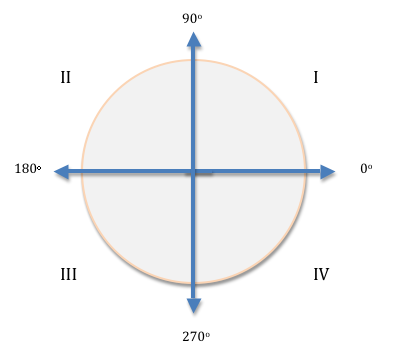Complete the program below so that it prompts the user for an angle in degrees, and prints out the quadrant in which the angle is located, represented by Roman numerals, as shown in the following figure.Notice that the value of the angle starts at zero degrees at the rightmost point of the circle, moving counterclockwise around the circle as the number of degrees grows. For instance, if the input angle has 100 degrees, it is in quadrant II, while an angle of 300 degrees is in quadrant IV and an angle of 45 degrees is in quadrant I. Each quadrant contains 90 degrees and starts at a multiple of 90. For example, 89 degrees is in quadrant I and 90 degrees is in quadrant II.

All the input angle values must be integers between 0 and 359. If the value of an angle falls outside this range, the word "Error" should be printed, and the program terminated.

Complete the following file: